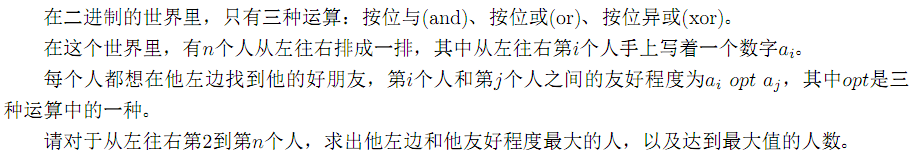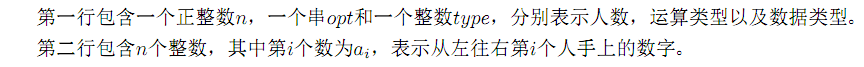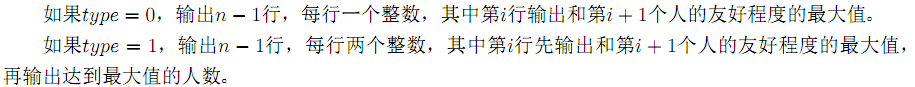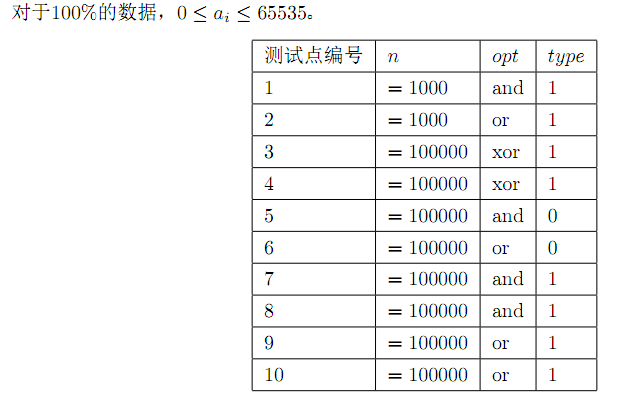# The binary world of Jzoj P4616

#### Description#### Input#### Output#### Sample Input

`5 and 13 5 2 7 1`

#### Sample Output

`1 12 15 11 3`

#### Data Constraint# Title Solution

• For ordinary violence, because ai is less than or equal to 2 ^ 16, violence can be added to O(1), while query O(2^16), which is obviously not allowed.
• Consider balancing the two operations. f[x][y] means that the first half is x, and the number of the second half and the maximum value of Y operation. g[x][y] records the number.
• When we add a number, we enumerate j and update f[a][j] by setting the first 8 bits of the number as a and the last 8 bits as b.
• When querying, set the first 8 bits of the number as a and the last 8 bits as B. we can enumerate I and update the answer with F [i] [b] | ((I ~ a) < 8).
• Complexity O(2^8*n)

# Code

``` 1 #include <cstdio>
2 #include <iostream>
3 #include <cstring>
4 using namespace std;
5 const int N=1e5+10,M=256;
6 int n,type,sum,ans,a[N],f[M][M],g[M][M];
7 char s;
8 int calc(int x,int y) { return s=='o'?x|y:(s=='a'?x&y:x^y); }
9 int main()
10 {
11     freopen("binary.in","r",stdin),freopen("binary.out","w",stdout);
12     scanf("%d%s%d",&n,&s,&type);
13     for (int i=1;i<=n;i++) scanf("%d",&a[i]);
14     for (int i=1;i<=n;i++)
15     {
16         int k=a[i]&255;
17         if (i>1)
18         {
19             sum=ans=0;
20             for (int j=0;j<=255;j++)
21                 if (g[j][k])
22                 {
23                     if ((f[j][k]|calc(j,a[i]>>8)<<8)==ans) sum+=g[j][k];
24                     else if ((f[j][k]|calc(j,a[i]>>8)<<8)>ans) ans=f[j][k]|calc(j,a[i]>>8)<<8,sum=g[j][k];
25                 }
26             if (type==1) printf("%d %d\n",ans,sum); else printf("%d\n",ans);
27         }
28         for (int j=0;j<=255;j++) if (f[a[i]>>8][j]==calc(j,k)) g[a[i]>>8][j]++; else if (f[a[i]>>8][j]<calc(j,k)) f[a[i]>>8][j]=calc(j,k),g[a[i]>>8][j]=1;
29     }
30 }```

Tags: PHP less

Posted on Fri, 01 Nov 2019 02:32:52 -0700 by TheIceman5